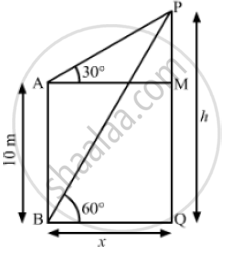# The Angle of Elevation of the Top of a Vertical Tower from a Point on Ground is 60° . from Another Point 10 M Vertically Above the First, Its Angle of Elevation is 30° .Find the Height of the Tower. - Mathematics

The angle of elevation of the top of a vertical tower from a point on the ground is 60° . From another point 10 m vertically above the first, its angle of elevation is 30° .Find the height of the tower.

#### SolutionLet PQ be the tower
We have,
AB =10m,MAP = 30° and ∠PBQ = 60°
Also, MQ = AB =10m
Let BQ = x and PQ = h
So, AM = BQ = x and PM = PQ - MQ =  h -10
In ΔBPQ,

 tan 60° = (PQ)/(BQ)

⇒ sqrt(3) = h/x

⇒ x = h/sqrt(3)                       ..............(1)

Now , in Δ AMP

 tan 30°  = (PM) /( AM)

⇒ 1/ sqrt(3) = (h-10) /x

⇒h sqrt(3)-10 sqrt(3)=x

⇒ h sqrt(3) - 10 sqrt(3) = h/ sqrt(3)                           [ Using (1)]

⇒ 3h  - 30=h

⇒ 3h - h = 30

⇒ 2h = 30

⇒ h 30/2

∴ h = 15 m

So, the height of the tower is 15 m.

Concept: Heights and Distances
Is there an error in this question or solution?
Chapter 14: Height and Distance - Exercises

#### APPEARS IN

RS Aggarwal Secondary School Class 10 Maths
Chapter 14 Height and Distance
Exercises | Q 21

Share